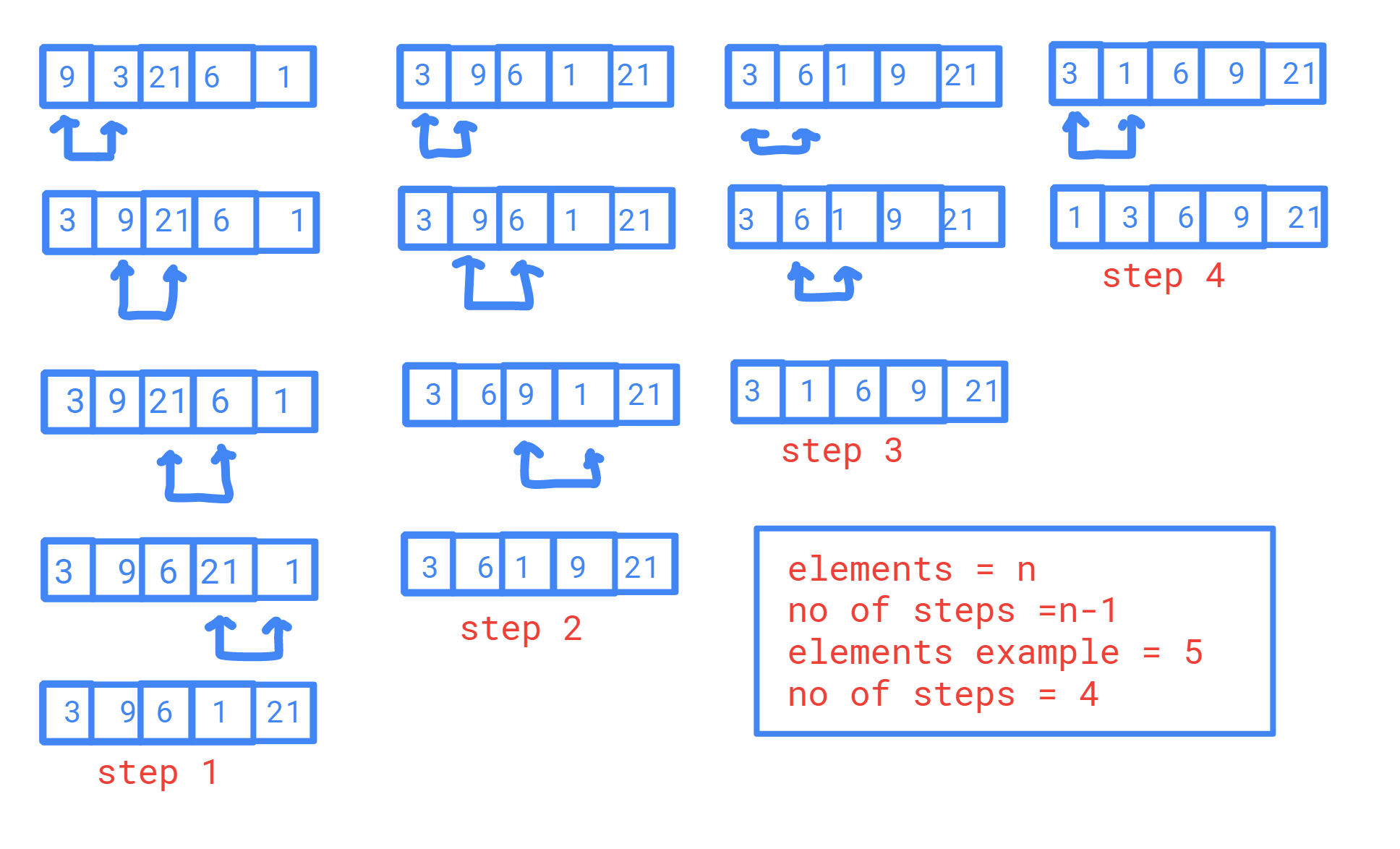# Bubble Sort

## Working of Bubble sort#

In bubble sorting, the comparison between two numbers takes place.there will be a sequence in comparison of the array elements. for ascending order first and second elements will be compared if the first element is greater than the second swapping of these array elements takes place .if the first element is smaller than second, they remain the same. next second element and third element will be compared and they are swapped if necessary .this process goes on until last and second the last element is compared and swapped.this is called bubble sort.

##### info

If the elements in the array are n .the process mentioned above should be repeated n-1times to get the required result.In the above example of Bubble sort

1. zeroth index element is 9, now 9 is compared with the next element, next 9 is greater than 3, swapping of 9,3 takes place
2. first index element is 9. this is compared with the second index element, 9 is smaller than 21, no swapping will be done
3. second index element is 21,21 is compared to the 6,21 is greater than 6, so swapping takes place
4. third index element is 21,21 is compared with the 1, (21 > 1 )so the swapping takes place
5. the first iteration is over , for the next iteration comparison will be done from the zeroth index but the last index is ignored because its already in the ascending order

## The time complexity of bubble sort#

• Worst Case Time Complexity [ Big-O ]: O(n2)
• Best Case Time Complexity [Big-omega]: O(n)
• Space Complexity: O(1)

## Program for Bubble sort#

#include<stdio.h>
int main()
{
int n,i,j,temp;
int arr;
printf("no of elements");
scanf("%d",&n);
for(i=0;i<n;i++)
{
printf("enter the %dst element",i+1);
scanf("%d",&arr[i]);
}
for(i=0;i<n;i++)
{
for(j=0;j<n-1;j++)
if(arr[j]>arr[j+1])
{
temp=arr[j];
arr[j]=arr[j+1];
arr[j+1]=temp;
}
}
printf("In ascending order: ");
for(i=0;i<n;++i)
printf("%d ",arr[i]);
return 0;
}

Last updated on by vishal devxo